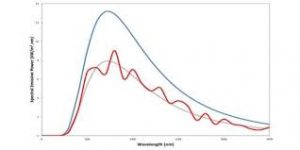## How to Calculate and Solve for Emissive Power | Radiation Heat TransferThe image above represents emissive power.

To compute for emissive power, two essential parameters are needed and these parameters are Area of Emitting Body (A) and Geometric Body Constant (F).

The formula for calculating emissive power:

Q = AF

Where:

Q = Emissive Power
A = Area of Emitting Body
F = Geometric Body Constant

Let’s solve an example;
Find the emissive power when the area of emitting body is 12 and the geometric body constant is 6.

This implies that;

A = Area of Emitting Body = 12
F = Geometric Body Constant = 6

Q = AF
Q = 12(6)
Q = 72

Therefore, the emissive power is 72 W.

Calculating the Area of Emitting Body when the Emissive Power and the Geometric Body Constant is Given.

A = Q / F

Where:

A = Area of Emitting Body
Q = Emissive Power
F = Geometric Body Constant

Let’s solve an example;
Find the area of emitting body when the emissive power is 32 and the geometric body constant is 8.

This implies that;

Q = Emissive Power = 32
F = Geometric Body Constant = 8

A = Q / F
A = 32 / 8
A = 4

Therefore, the area of emitting body is 4.